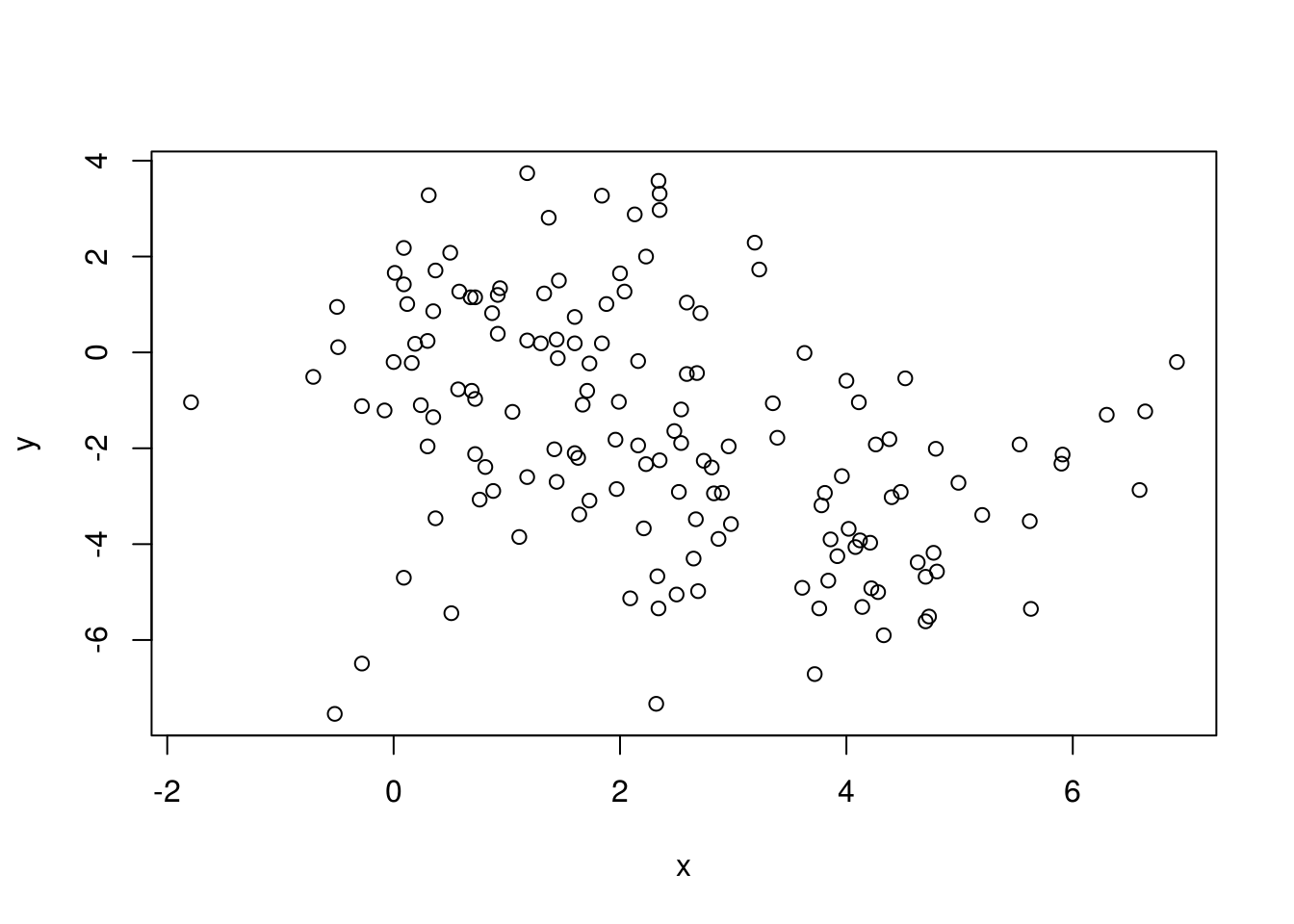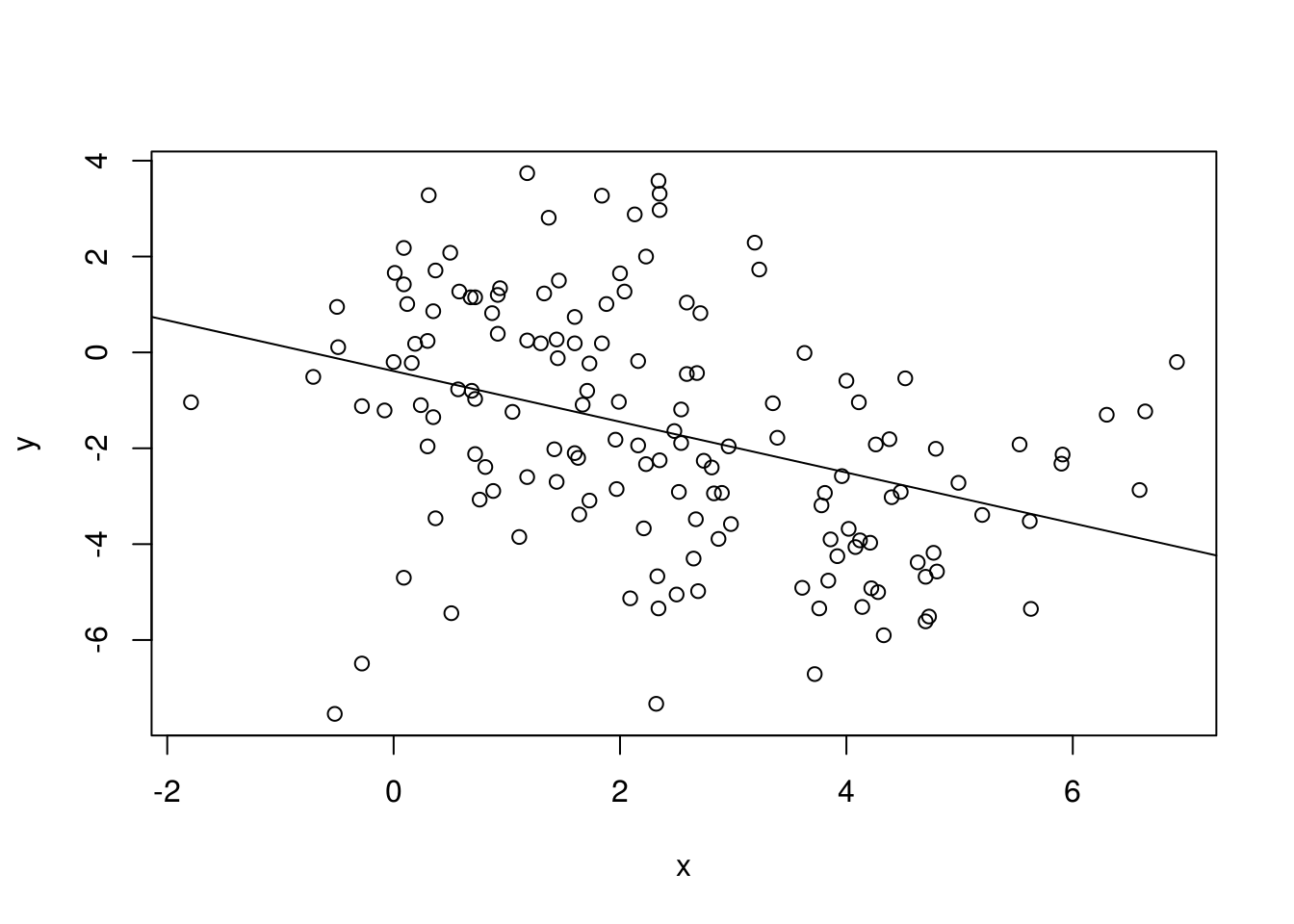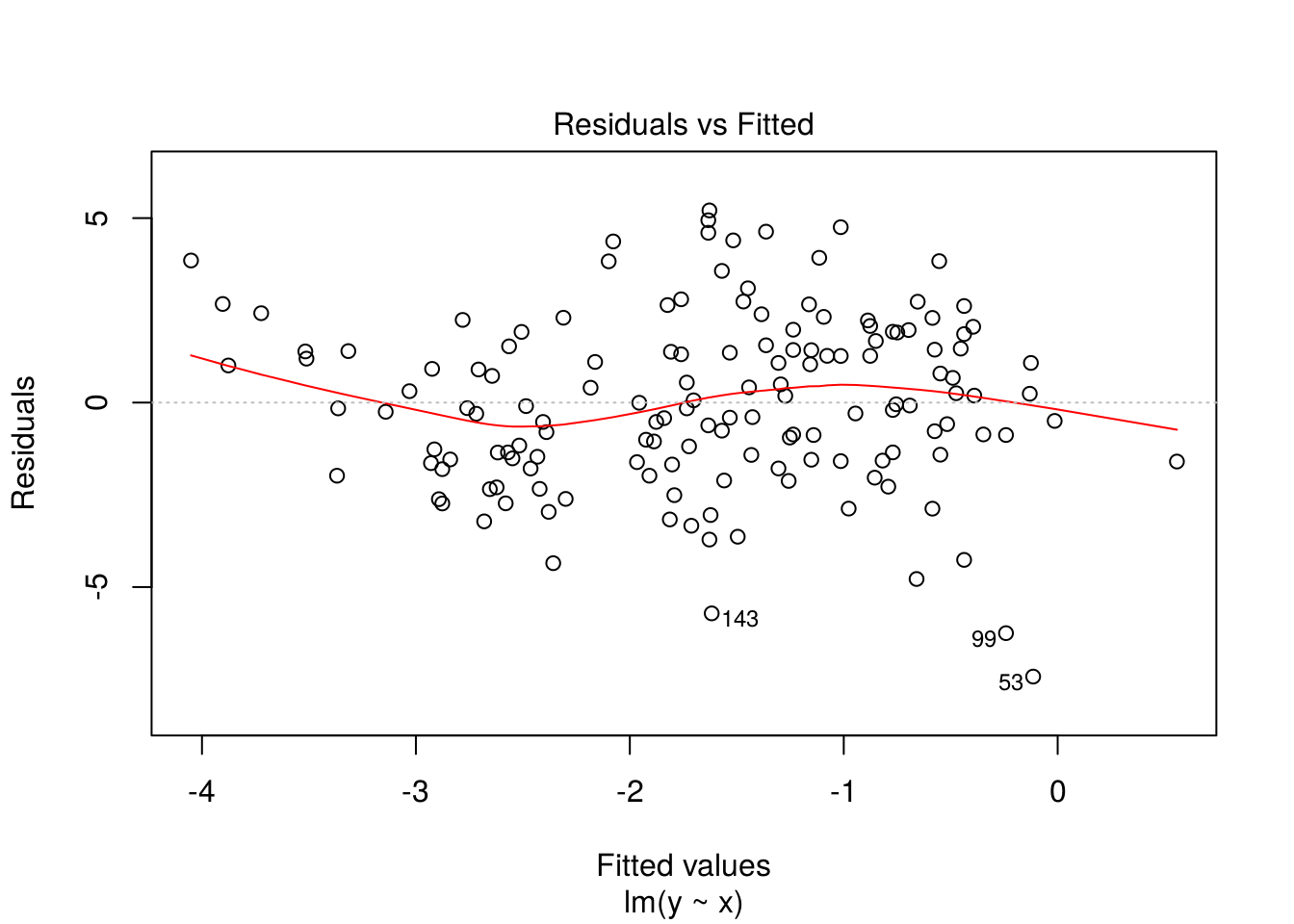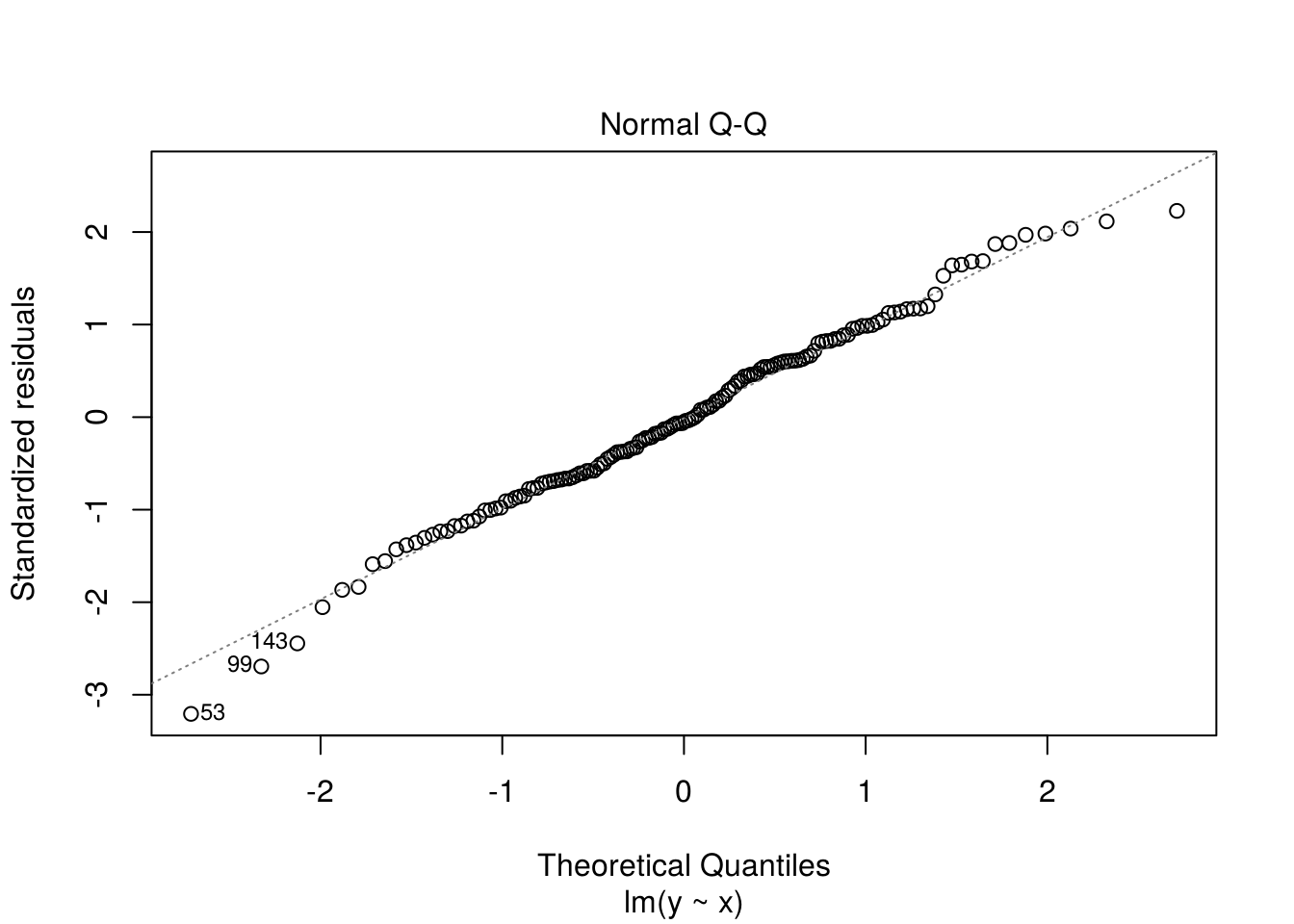# Lecture 5

## Linear model as a basic exemplar

A template for lots of models for dependence of a variable (the response variable) upon other variables (the explanatory or predictor variables): multiple linear regression.

mymodel <- lm(my.response ~ my.predictor1 + my.predictor2, data = mydata)

The ingredients here are:

• mymodel: the name I am giving to the resulting model object that will be stored in my current R workspace
• mydata: the data frame where lm() should look first for the variables used in my model
• my.response: the ‘response’ or ‘outcome’ variable. (What SPSS would often call the dependent variable.)
• my.predictor1 etc.: the explanatory or predictor variables for the model
• ~: means roughly is modelled as a function of
• +: takes the effects of my.predictor1 and my.predictor2 to be additive
• lm(): calls the R function needed to specify and fit a linear model by least squares.

In mathematical notation, this model looks like $y_i = \beta_0 + \beta_1 x_{i1} + \beta_2 x_{i2} + e_i \qquad(i=1,...n)$ where $$e_i$$ is the ‘error’. (By default, the errors are assumed to have constant variance.)

## Linear model: Other commonly used arguments to lm()

The above template can be modified by further arguments to lm(). The most commonly used of these are:

• weights: specify a vector of case-weights. Useful for situations where error variances are known to be unequal but their ratios are known.
• e.g., measurements come from 2 different instruments, one of which is known to have 10% more variance than the other: weights = 1/(1 + (0.1 * (instrument == "less_accurate")). The weights should be proportional to 1/variance.
• subset: specify a subset of the data to be used (the remainder being ignored).
• For example, subset = (gender == "female")

## A model formula can include all sorts of predictor!

Examples are:

• factors: categorical predictors such as gender, race, …

• interactions: if the effect of p1 might be different at different levels of p2, consider the interaction p1 : p2

• polynomials: for example, to fit a quadratic $$y = x + x^2 + e$$, use as model formula y ~ x + I(x^2). The wrapper I() is needed here because otherwise R would interpret x^2 to mean the same thing as x:x (which by convention is the same as just x).

• spline functions: various forms of spline function are implemented. Among the simplest to use are B-splines and natural cubic splines. With library(splines) loaded, such spline functions can be used in a model formula via terms such as bs(x) and ns(x).

## Standard operations on a model object

What we should want to know first, after fitting a model to data, is:

• does the model fit the data?
• can the model be improved?

The key here is to look (in whatever ways we can think of) at the model’s residuals. The plot() function applied to a model object is a good place to start with this. If the model is a good model and cannot be improved, then it should not be possible to find any systematic patterns in the residuals.

Once a model is found that fits the data well, interest then shifts to:

• What does the model say about my research question(s)? This typically is answered through:
• parameter estimates, and confidence intervals for the parameter(s) of interest
• hypothesis tests

Part of the art of modelling is to try to encompass your research question in a model parameter, or at most a small number of model parameters.

## Beyond linear models

Linear models will not be appropriate for some commonly encountered types of response variable, including:

• categorical response variables (use logistic regression, probit regression, etc.; and multi-category versions of these)
• counts (typically use log-linear models based on the Poisson or negative binomial distributions)
• failure-time or ‘survival’ data (use proportional hazards models or accelerated failure-time models).

And sometimes linearity just won’t do the job, even on another scale such as logit or probit, because the effects being studied are inherently non-linear.

R provides a huge array of alternative modelling possibilities.

These include:

• glm() for all generalized linear models (includes logistic regression, log-linear models, etc.)
• gam() (from the recommended mgcv package) which fits generalized additive models, very flexible models based on spline functions
• the survival package (for time-to-event data, typically with censoring)
• the nls() function for least-squares fitting of nonlinear regression models, and the gnm package for generalized nonlinear models

— and many, many more!

## A small (artificial) example

Just to demonstrate that even seemingly simple linear models need care, in formulation and interpretation.

Here are some data:

with(mydata, plot(x, y))We are interested in how $$y$$ depends on $$x$$.

Let’s fit a linear model:

model1 <- lm(y ~ x, data = mydata)
with(mydata, plot(x, y))
abline(model1)Let’s look next at the residuals:

plot(model1)